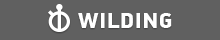GMAT Question of the Day - Daily to your Mailbox; hard ones only

 It is currently 26 Jun 2019, 11:17GMAT Club Daily Prep

Thank you for using the timer - this advanced tool can estimate your performance and suggest more practice questions. We have subscribed you to Daily Prep Questions via email.

Customized
for You

we will pick new questions that match your level based on your Timer History

Track

every week, we’ll send you an estimated GMAT score based on your performance

Practice
Pays

we will pick new questions that match your level based on your Timer History

Not interested in getting valuable practice questions and articles delivered to your email? No problem, unsubscribe here.In a certain class, 3 out of 24 students are in student organizations.

Author Message
TAGS:

Hide Tags

Senior RC ModeratorV
Status: ----__----___-----____-----
Joined: 02 Nov 2016
Posts: 2769
Location: Pakistan
GPA: 3.39
In a certain class, 3 out of 24 students are in student organizations.  [#permalink]

Show Tags00:00

Difficulty:15% (low)

Question Stats:85% (00:34) correct15% (00:55) wrongbased on 36 sessions

HideShow timer StatisticsIn a certain class, 3 out of 24 students are in student organizations. What is the ratio of students in student organizations to students not in student organizations?

A. $$\frac{1}{8}$$

B. $$\frac{1}{7}$$

C. $$\frac{1}{6}$$

D. $$\frac{1}{5}$$

E. $$\frac{1}{4}$$

Source: Kaplan GMAT Math Workbook - Sixth Edition
Page# 305

_________________
New Project RC Butler 2019 - Practice 2 RC Passages Everyday
Final days of the GMAT Exam? => All GMAT Flashcards.
This Post Helps = Press +1 Kudos
Best of Luck on the GMAT!!
ManagerG
Joined: 17 Mar 2015
Posts: 88
Re: In a certain class, 3 out of 24 students are in student organizations.  [#permalink]

Show Tags

In a certain class, 3 out of 24 students are in student organizations. What is the ratio of students in student organizations to students not in student organizations?

A. $$\frac{1}{8}$$

B. $$\frac{1}{7}$$

C. $$\frac{1}{6}$$

D. $$\frac{1}{5}$$

E. $$\frac{1}{4}$$

Source: Kaplan GMAT Math Workbook - Sixth Edition
Page# 305

In a certain class, 3 out of 24 students are in student organizations. What is the ratio of students in student organizations to students not in student organizations?
B should be OA
3/21
VPP
Joined: 31 Oct 2013
Posts: 1376
Concentration: Accounting, Finance
GPA: 3.68
WE: Analyst (Accounting)
Re: In a certain class, 3 out of 24 students are in student organizations.  [#permalink]

Show Tags

In a certain class, 3 out of 24 students are in student organizations. What is the ratio of students in student organizations to students not in student organizations?

A. $$\frac{1}{8}$$

B. $$\frac{1}{7}$$

C. $$\frac{1}{6}$$

D. $$\frac{1}{5}$$

E. $$\frac{1}{4}$$

Source: Kaplan GMAT Math Workbook - Sixth Edition
Page# 305

let the total students be 24.

students involved in organization = 3

students are not involved in org = 21

Ratio : 3/21 = 1/7

ManagerB
Joined: 14 Feb 2016
Posts: 71
Re: In a certain class, 3 out of 24 students are in student organizations.  [#permalink]

Show Tags

B.

3/24 - in student organization. Implies that 21/24 are not in a student organization.

Ratio of students in to students not in:

3/21 --> simplified to --> 1/7

_________________
Please provide kudos if you liked my post! I would really like to use the PDF file!!
ManagerS
Joined: 24 Nov 2018
Posts: 109
Location: India
GPA: 3.27
WE: General Management (Retail Banking)
Re: In a certain class, 3 out of 24 students are in student organizations.  [#permalink]

Show Tags

In a certain class, 3 out of 24 students are in student organizations. What is the ratio of students in student organizations to students not in student organizations?

A. $$\frac{1}{8}$$

B. $$\frac{1}{7}$$

C. $$\frac{1}{6}$$

D. $$\frac{1}{5}$$

E. $$\frac{1}{4}$$

Source: Kaplan GMAT Math Workbook - Sixth Edition
Page# 305

No of students in student organization=3
No of students not in student organization=24-3=21
Ratio=3/21=1/7
IMO, Option B
_________________
Kudos encourage discussions. Share it to amplify collective education!Re: In a certain class, 3 out of 24 students are in student organizations.   [#permalink] 26 Dec 2018, 18:24
Display posts from previous: Sort by

In a certain class, 3 out of 24 students are in student organizations.